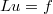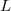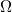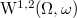﻿

### Existence and approximation of solutions for a class of degenerate elliptic equations with Neumann boundary condition

#### Abstract

In this work we study the equation$Lu=f$, where$L$ is a degenerate elliptic operator, with Neumann boundary condition in a bounded open set${\Omega}$. We prove the existence and uniqueness of weak solutions in the weighted Sobolev space${\mathrm{W}}^{1,2}(\Omega , \omega)$ for the Neumann problem. The main result establishes that a weak solution of degenerate elliptic equations can be approximated by a sequence of solutions for non-degenerate elliptic equations

DOI Code: 10.1285/i15900932v40n2p63

Keywords: Neumann problem; weighted Sobolev spaces

Full Text: PDF